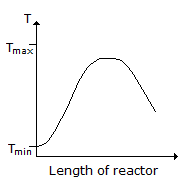# Chemical Engineering - Chemical Reaction Engineering

### Exercise :: Chemical Reaction Engineering - Section 9

41.

For a __________ order chemical reaction,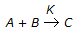, the fractional conversion of reactant 'A' is proportional to time.

 A. zero B. first C. second D. third

Explanation:

No answer description available for this question. Let us discuss.

42.

Velocity of a chemical reaction

 A. decreases with increase in temperature. B. increases with increase of pressure of reactants for all reactions. C. decreases with increase of reactant concentration. D. none of these.

Explanation:

No answer description available for this question. Let us discuss.

43.

Backmixing is most predominant in

 A. a well stirred batch reactor. B. a plug-flow reactor. C. a single CSTR. D. CSTR's connected in series.

Explanation:

No answer description available for this question. Let us discuss.

44.

If helium is introduced in a reactor containing O2, SO2 and SO3 at equilibrium, so that total pressure increases while volume and temperature remains constant. In this case the dissociation of SO3 (as per Le Chatlier principle)

 A. decreases B. increases C. remains unaltered D. changes unpredictably.

Explanation:

No answer description available for this question. Let us discuss.

45.

Consider a reversible exothermic reaction in a plug flow reactor. The maximum and minimum permissible temperatures are Tmax and Tmin respectively. Which of the following temperature (T) profiles will require the shortest residence time to achieve the desired conversion.

 A.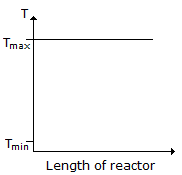B.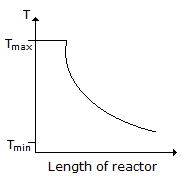C.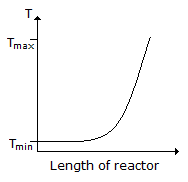D.